# Using Genetic Algorithms to Efficiently Trade the Wheel (Python)

The wheel is a loss-limited option selling strategy that is very effective at generating income in a portfolio. Typically when trading the wheel, stocks with weekly options are preferred since they fetch higher premiums (percentage-wise) than monthly options or LEAPS. However, due to the high number of stocks on US stock exchanges offering weekly options and the differences in volatility and price, it’s challenging to find an optimal set of stocks to sell puts against that will maximize your collateral usage and income while minimizing the risk taken due to volatility.

Optimization problems like this, wherein some variables are maximized while others are minimized, are in a class of problems known as multi-objective optimization. Unfortunately, for any nontrivial problem, there isn’t a single solution that perfectly optimizes all of the optimization objectives, rather there is a set of solutions known as Pareto optimal solutions which are all considered equally good. These solutions are all considered optimal since one objective cannot be improved without simultaneously worsening another.

The genetic algorithm is a biology-inspired algorithm that “evolves” a near-optimal solution. Genetic algorithms have been applied to many different problems including multi-objective optimization via algorithms such as SPEA, SPEA2, PAES, NSGA, NSGA-II, and NPGA2, to name a few. Solving constrained multi-objective optimization problems using genetic algorithms is a vast field of research and resources abound online for readers interested in pursuing that further.

In this post, measurements of a stock’s volatility, the premium gained by selling weekly put options, and the availability of collateral are used as the inputs to a genetic algorithm’s fitness function. The solution is evolved for a pre-determined number of generations and the best solution is displayed. Ideally, a Pareto optimal solution will be provided that offers a portfolio in which income is maximized, volatility (i.e. risk, especially in this case) is minimized, and a constraint based on the available collateral is satisfied. The problem is more formally explained and solved below.

## The Wheel

For the uninitiated reader, more information about options and option strategies abound on the internet. For those wanting a really excellent entry point into the world of options, I personally recommend the book Understanding Options by Michael Sincere.

The wheel is a loss-limited, income-generating stock options selling strategy that allows a trader to earn premiums off of collateral available in their account. The strategy starts by selling cash-covered out-of-the-money puts in an account. The trader must have enough collateral to purchase 100 shares (per option sold) of the underlying stock at the designated strike price if they are assigned the shares. Typically, shares are only assigned if the price of the stock falls below the strike price on the put option. The trade generates income since they receive premium for taking the risk of being assigned the shares if the price were to drop. For example, if, at the time of writing, a trader had $1,200 in their account they could use this cash as collateral to sell an out-of-the-money put option on General Electric (GE) at the$12 strike price and March 19, 2021 expiration for about $16. This$16 is the premium earned and now belongs to the investor, it can be withdrawn, reinvested, or otherwise used.

Now, using the example above, assume the stock price of General Electric falls below $12 per share, say$11.75, and stays there on expiration day (March 19th). The trader is now obligated to purchase 100 shares of GE at $12 per share so the$1,200 of cash in their account which was used as collateral is used to purchase the General Electric stock. Notice that the market price is lower than the strike price by $0.25 which, essentially, gives the trader a$25 dollar loss less the $16 in premium earned for a total capital loss of$9. However, when trading the wheel small capital losses are somewhat inconsequential due to the other side of the strategy which is selling covered call options.

Once the shares are in the trader’s account they can now be used as collateral when selling a call option. Selling this type of option obligates the trader to sell his shares at a specified price (the strike price) on a specified date (the expiration date). When the trader is forced to sell their shares the shares are said to be “called” away. Typically, the shares are only called if the market price is above the strike price on expiration. Sticking with the General Electric example the trader now owns 100 shares of GE with a per-share cost basis of $12 and a market price of$11.75. The week after being assigned these shares (assignment usually happens Friday evening) the trader now sells a covered call, again at the $12 strike price and the nearest expiration (the following Friday since GE has weekly options). Now the shares only leave the trader’s account if they rise above$12/share. Note that this is the same price that the shares were assigned so if/when the shares are called, the capital loss is negated and the total gain is the premium collected from selling the put and call options. In general, the total gain for this strategy is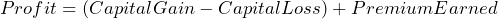In the case that the stock is neither assigned (for covered puts) or called (for covered calls), the trader will wait until the option expires and sell another put using their collateral or another call using the shares currently in their portfolio. Below is a simple flowchart for the decision-making behind this options strategy.

The wheel is a very powerful strategy for generating income on any portfolio. Provided here is an example of a more or less ideal situation but the strategy does come with many risks. The interested investor is encouraged to find more in-depth resources on this strategy (which are out of the scope of this post) and to consider and analyze the potential risks of trading options in this way. The GE example is not intended to be a tutorial or instructions on trading the wheel and the description here is in no way investment advice.

## Genetic Algorithms (GAs)

Genetic algorithms (GAs) are algorithms that search by simulating evolution. That is, searching is done by approximating biological functions from natural selection such as reproduction and mutation. Each individual (or genome) in a genetic algorithm’s population is a potential solution to the problem being solved. Using this genome, a fitness value can be assigned to each individual. Individuals with the highest fitness are, typically, most likely to reproduce and create new individuals for a future population in the algorithm. The fitness function, i.e. the function that assigns the fitness value to a particular genome, is vitally important for the success of the genetic algorithm. Other variable processes in the algorithm include the selection of individuals to reproduce, the way in which individuals reproduce, and when and how genomes are mutated after reproduction.

The different ways that genetic algorithms can be implemented and how selection, reproduction, and mutation can be performed are outside the scope of this post. There are many resources available that discuss and describe many of the popular ways to handle these biological processes (many will do a far better job than me at explaining them). Personally, I’m partial to Stephen Marsland’s book Machine Learning An Algorithmic Perspective which is sometimes available for cheap on ThriftBooks and usually available on Amazon for a reasonable price (~$30). In the case of the implementation for this project the genome was a sequence of 1’s and 0’s where a 1 represented using a security for the wheel and a 0 represented not using the security. There were about 300 securities under consideration since only stocks with weekly options and within a certain price range (i.e. stocks that we actually have enough collateral for) were considered. Therefore, there are about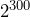unique solutions to the problem. Of course, not all of these solutions are feasible as most of them would use way more collateral than is available but each could potentially be created/generated by the genetic algorithm. Note here that with so many unique solutions it would be impossible to, within a reasonable amount of time, find a set of optimal solutions, i.e. solutions that maximize income and minimize risk. These types of search problems (those with large search spaces) are a quintessential application of genetic algorithms. ## Implementation The tickers used are provided by CBOE and are for stocks that offer weekly options. The full list can be found here. In my personal account used for trading the wheel, my goal is to earn ~1% per week off of the collateral available. This provides a steady stream of income (weekly) and reduces risk by limiting exposure to market fluctuations due to the short time period (usually 4 days). Additionally, I’ve found that it’s usually easier to meet the 1% per week goal with weekly options when compared to selling monthly options with the goal of earning 4% of the collateral on the trade. Due to this minimum income constraint, the algorithm produces an output that includes the income received as a percentage of the collateral available. It’s important to note that this algorithm is only used for selling put options when beginning the wheel as there is no choice of stock when selling call options with this strategy. This is because the trader is forced to sell call options on the shares they were assigned. The implementation of the genetic algorithm was done in Python using the “Distributed Evolutionary Algorithm in Python” (DEAP) framework. Pandas and AllyInvest.py are also required to run the algorithm. These dependencies can be installed via pip with the following command: pip install deap pandas AllyInvestPy  API keys for AllyInvest.py are also required which requires an Ally Invest account. For those wanting to run the algorithm and not have an Ally Invest account, the data_fetcher.py implementation can be updated to use a different data source to fetch the stock and options data. ### Fetching Security and Option Data To begin, a class was implemented to filter the tickers based on the collateral available and fetch the necessary information for each eligible stock. This information was gathered from Ally Invest using the AllyInvest.py library. import pandas as pd from ally import AllyAPI from ally.requests import QuotesRequest import datetime import time class DataFetcher(object): def __init__(self, ticker_filename, expiration_date, collateral, strikes_out=1): """ ticker_filename -> filename for tickers to be considered (csv) expiration_date -> expiration date of the options considered collateral -> amount of funds available as collateral strikes_out -> how many strikes below current price - note that this is only for Puts for Calls options are traded on the assigned shares """ self.filename = ticker_filename self.expiration = expiration_date self.strikes_out = strikes_out self.collateral = collateral self.CONSUMER_KEY = "YOUR CONSUMER KEY" self.OAUTH_TOKEN = "YOUR OAUTH TOKEN" self.OAUTH_SECRET = "YOUR OAUTH SECRET" self.ally = AllyAPI(self.OAUTH_SECRET, self.OAUTH_TOKEN, self.CONSUMER_KEY, response_format='json') def fetch_data(self): ticker_csv = pd.read_csv(self.filename) # get stock quotes for all tickers; keep price, symbol, and beta measurement data = [] tickers_per_request = 400 # before maxing out URL length tickers = ticker_csv["Ticker"].tolist() # chunk data based on tickers_per_request variable tickers = [tickers[x:x+tickers_per_request] for x in range(0, len(tickers), tickers_per_request)] for ticker_list in tickers: quote_request = QuotesRequest(symbols=ticker_list) response = quote_request.execute(self.ally) for quote in response.get_quotes(): # bad fetch or too expensive or bankrupt - ignore security if quote.symbol == 'na' or float(quote.last)*100 > self.collateral \ or float(quote.bid) == 0 or float(quote.ask) == 0 or quote.beta == '': continue data.append([quote.symbol, ((float(quote.ask) + float(quote.bid))/2.0), float(quote.beta)]) # get available strike prices for all tickers (ally.get_options_strikes(symbol)) count = 0 for dp in data: if count == 100: # this isn't the rate limit but there are other processes using the API print("Rate limit reached, sleeping for 15 seconds...") time.sleep(15) count = 0 js = self.ally.get_options_strikes(dp) # unfortunately, this can't take multiple tickers strikes = js['response']['prices']['price'] # get index of nearest strike less than current price idx = next((x for x, val in enumerate(strikes) if float(val) > dp), 0) - self.strikes_out if idx < 0: dp.append(0) continue strike_price = float(strikes[idx]) dp.append(strike_price) count += 1 tickers_per_request = 100 tickers = ticker_csv["Ticker"].tolist() tickers = [tickers[x:x+tickers_per_request] for x in range(0, len(tickers), tickers_per_request)] for ticker_list in tickers: putcall, dates = [], [] for _ in range(len(ticker_list)): putcall.append("p") dates.append(self.expiration) tickers, strikes = [], [] for ticker in ticker_list: for dp in data: if dp == ticker: tickers.append(ticker) strikes.append(dp) break quote = self.ally.get_option_quote(tickers, dates, strikes, putcall)['response']['quotes']['quote'] for q in quote: for dp in data: if dp == q['undersymbol']: dp.append(((float(q['ask']) + float(q['bid']))/2)) break return pd.DataFrame(data, columns=['symbol', 'price_mid', 'beta', 'option_strike', 'option_income']) if __name__ == '__main__': fetcher = DataFetcher('weekly_option_tickers.csv', datetime.datetime(2021, 3, 5), 250000, strikes_out=1) data = fetcher.fetch_data()  The if __name__ == ‘__main__’ is included above so that the data fetching capabilities can be tested as a standalone process by running python3 data_fetcher.py. Notice that the data fetching class takes a strikes_out variable. This variable is used to find the strike price we want to sell the option at and is the number of strike prices away from the current stock price. For example, for near-the-money strike prices, GE trades in$0.50 increments ($12.50,$13.00, $13.50, etc.) At the time of writing, GE is trading around$13.34 per share so, when selling an out-of-the-money put our strike price choices are $13.00,$12.50, $12.00, etc. If the strikes_out variable is set to 1, then$13.00 will be the strike price of the option sold. If strikes_out is set to 2, $12.50 will be the strike price of the option. When selling out-of-the-money puts, the further the strike price is from the stock price the less likely we are to be assigned the shares of the stock on expiration. The trade-off is that further out-of-the-money strikes command less premium so less income is earned. In sum, the strikes_out variable represents the number of strike prices out-of-the-money that the options traded will use. This data fetching class starts by fetching all of the data from the CBOE weekly options list. If the data is unavailable, the data request fails, or the security is too expensive (i.e. we don’t have enough collateral to sell puts on it) then the security is ignored. For all of the acceptable securities, options information is fetched and a Pandas data frame is created storing the ticker symbol, the stock price, the stock’s beta value, the option’s strike price (for collateral requirements), and the option’s premium price (1/100th of the income received when selling the option). ### Creating a Population for the GA This project assumes that relatively small portfolios will be used, i.e. those with collateral under$20,000. If there are any hedge fund managers reading this and wanting to apply this algorithm you’ll have to reach out to me for help modifying the population generation. Because of this assumption, creating population individuals with many 1’s in the bitstring prevents the algorithm from ever (within a reasonable amount of time) reaching a feasible pseudo-optimal portfolio. Even generating 1’s and 0’s with equal probability will produce portfolios requiring hundreds of thousands of dollars in collateral. To fix this, a custom individual generation function was implemented to create a “sparsely-populated” individual (that is, one with very few 1’s).

def random_sample(pop):
# 95% chance of 0, 5% chance of 1
population_values = *95 + *5
random.shuffle(population_values)

return random.sample(pop, 1)

In the function above, a list of 100 values being either 0 or 1 is generated. Of these values, 95 of them are 0’s, and 5 of them are 1’s. When generating the individual a random value is selected from this list so that each bit in the individual’s bitstring representation is a 1 with 5% probability and a 0 with 95% probability. This will create an individual in which few securities will be used when trading the wheel keeping collateral requirements low.

### Evaluating an Individual’s Fitness

As previously mentioned, the correct fitness function is essential for a well-performing genetic algorithm. In this case, two variables are being optimized (income and risk [beta]) and there is one constraint. Therefore, a good fitness function will include all of these variables and prevent any one of them from overpowering the others. One exception to the overpowering rule is actually used in the collateral constraint. Since it is critical that the collateral used remains under the collateral available an individual’s fitness is reduced by the amount of collateral used over the collateral available. This way, the larger the difference between its used and available collateral the less likely an individual will be to reproduce.

Another thing to note is the incorporation of the beta measure for the stocks in the fitness function. Beta shouldn’t necessarily be minimized since large negative beta values still imply high volatility just that the swings are typically opposite of “the market”. One way to think about beta is that it’s the percent change expected for a 1% change in the market. That is, if a stock’s beta is 0.75 then a 1% change in the market would illicit a 0.75% change in the stock. Similarly, a -0.75% beta implies that a 1% change in the market will illicit a -0.75% change in the stock price. Of course, this isn’t always going to be the case (you also have to define “the market”). The mathematical definition accentuates this reliance on the covariance of returns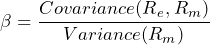where R_e is the return on the individual stock and R_m is the return of the overall market. In this application, we don’t really care if the beta value is positive or negative but we do want the securities underlying the options we trade to have low volatility to reduce the likelihood of assignment. Therefore, ideal values of variance are between -1 and 1 as values in this range imply that the stock typically moves less than the market (because they are fractional values). In the fitness function for this GA the beta’s distance from 1 is used rather than the beta itself. That is, beta for the fitness equation is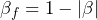the average of the beta values defined in this way for each selected stock is used in the fitness function.

The fitness function is provided below.

# globals so they can easily be accessed everywhere
# (these should never be changed)
collateral = 11500
stocks = None

def evaluate(individual):
score = 0
used_coll, income, beta = 0, 0, 0
count = 0
for i in range(len(individual)):
if individual[i] == 1:
stock = stocks.iloc[i]
used_coll += stock["option_strike"]*100
beta += (1 - abs(stock["beta"])) # distance from 1: low = good
income += stock["option_income"]   # maximize income
count += 1  # average beta is important, not total beta
if count > 0:
beta = beta/count   # count = number of stocks => average beta
# score is designed to be minimized
score = 0.1*income + beta
if used_coll > collateral:
score -= used_coll - collateral
return score,

Since income is usually much higher than the average beta value it is divided by 10 so that it doesn’t overpower the fitness equation. All said and done the fitness function is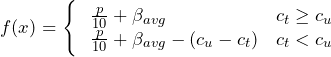where c_u is the amount of collateral used, c_t is the total amount of collateral available, and p is the premium collected from selling options on the selected stocks.

### Results

Below are some example portfolios when running the algorithm for different numbers of generations, amounts of collateral, and initial likelihoods of trading options on a stock (i.e. changing the genome generation function to generate more or fewer 0’s). For these, DEAP’s eaSimple algorithm was used with a mating probability (cxpb) of 50%, a mutation probability of 20%, and a population of 300 individuals. Note that this data is only accurate at the time of running the algorithm.

Running the algorithm for 1,000 generations with an initial probability of using a security set to 7%, that is the genome generation function looks like this

def random_sample(pop):
# 93% chance of 0, 7% chance of 1
population_values = *93 + *7
random.shuffle(population_values)

return random.sample(pop, 1)

and when using $12,500 as collateral gives the following portfolios (by way of example, each run will create a different portfolio, probably). These two portfolios accentuate a problem in multi-objective optimization. Notice that the first portfolio has a high beta (higher than we’d like) but also a more reasonable amount of income while the algorithm favored a low beta and a low income in the second portfolio. The first portfolio prioritizes income over risk and the second prioritizes risk reduction over income. Both of these strategies will lead to high values for the fitness function. Next, the algorithm was run for the same parameters as the previous set but now the GA is given 5,000 generations to find an optimal portfolio. Theoretically, giving the algorithm more time to find a portfolio should produce better portfolios as more of the search space should be checked. Using more population members can also help with this. The trade-off is an increase in run time. In this portfolio, good values for risk and income are found but the individual leaves some collateral on the table. Improving the fitness function to penalize individuals that use too little collateral would help circumvent this issue. However, the portfolios tend to be pretty good without this addition and smaller portfolios should have less income which is penalized by the fitness function. Running the algorithm for even more generations might also produce a more optimal portfolio. The final run of the GA uses 2,000 generations and$25,000 of available collateral. Because of this, the initial probability of using a stock in an individual’s wheel portfolio is increased to 8%.

The main reason for doing this was to see how well the algorithm scales to larger portfolios. Increasing the probability of generating a 1 in the genome generation function should help with even larger portfolios as more securities will need to be traded to use more collateral. However, generating a portfolio for small amounts of collateral and selling multiple put options on each security is also a viable strategy.

## Conclusion

In this post, a GA was discussed and implemented for trading an income-generating options selling strategy known as the wheel. The algorithm was run for different sets of parameters and a few potential portfolios were presented. The full code for the project is available at the end of this post.

As seen here, this is algorithm is a viable tool to be added to the options trader’s toolkit however it should be noted that it’s not advisable to trade options for underlying stocks that you wouldn’t consider owning (especially in the case of the wheel due to potential assignments). Research should still be conducted on the stocks you’re planning to wheel to help reduce the risk of the portfolio (as is the case with any portfolio).

There are some potential improvements to this tool. First, the GA only considers selling a single put option for each underlying security. Changing the bit string representation of each individual to a string of small numbers, e.g. 0’s, 1’s, 2’s, and 3’s, where the number represents how many options to sell for each security might produce more interesting results, but note that it would lower diversification. Additionally, there are GA designed for more or less this type of optimization. I believe the most prominent are the different flavors of the NSGA algorithm. Using one of these optimization algorithms over the simple GA used here might improve the portfolios as well. Performance could likely be enhanced by fine-tuning the cxpb, mu, population size, and generation parameters too.

## Full Code

### data_fetcher.py

import pandas as pd
from ally import AllyAPI
from ally.requests import QuotesRequest
import datetime
import time

class DataFetcher(object):
def __init__(self, ticker_filename, expiration_date, collateral, strikes_out=1):
"""
ticker_filename -> filename for tickers to be considered (csv)
expiration_date -> expiration date of the options considered
collateral -> amount of funds available as collateral
strikes_out -> how many strikes below current price
- note that this is only for Puts for Calls options are
"""
self.filename = ticker_filename
self.expiration = expiration_date
self.strikes_out = strikes_out
self.collateral = collateral

self.CONSUMER_KEY = "your Ally Invest consumer key"
self.OAUTH_TOKEN = "your Ally Invest OAUTH token"
self.OAUTH_SECRET = "your Ally Invest OAUTH secret"

self.ally = AllyAPI(self.OAUTH_SECRET, self.OAUTH_TOKEN, self.CONSUMER_KEY, response_format='json')

def fetch_data(self):

# get stock quotes for all tickers; keep price, symbol, and beta measurement
data = []
tickers_per_request = 400
tickers = ticker_csv["Ticker"].tolist()
tickers = [tickers[x:x+tickers_per_request] for x in range(0, len(tickers), tickers_per_request)]
for ticker_list in tickers:
quote_request = QuotesRequest(symbols=ticker_list)
response = quote_request.execute(self.ally)
for quote in response.get_quotes():
# bad fetch or too expensive or bankrupt
if quote.symbol == 'na' or float(quote.last)*100 > self.collateral \
or float(quote.bid) == 0 or float(quote.ask) == 0 or quote.beta == '':
continue

# get available strike prices for all tickers (ally.get_options_strikes(symbol))
count = 0
for dp in data:
if count == 100:    # this isn't the rate limit but there are other processes using the API
print("Rate limit reached, sleeping for 15 seconds...")
time.sleep(15)
count = 0
js = self.ally.get_options_strikes(dp)   # unfortunately, this can't take multiple tickers
strikes = js['response']['prices']['price']
# get index of nearest strike less than current price
idx = next((x for x, val in enumerate(strikes) if float(val) > dp), 0) - self.strikes_out
if idx < 0:
dp.append(0)
continue
strike_price = float(strikes[idx])
dp.append(strike_price)
count += 1

tickers_per_request = 100
tickers = ticker_csv["Ticker"].tolist()
tickers = [tickers[x:x+tickers_per_request] for x in range(0, len(tickers), tickers_per_request)]
for ticker_list in tickers:
putcall, dates = [], []
for _ in range(len(ticker_list)):
putcall.append("p")
dates.append(self.expiration)

tickers, strikes = [], []
for ticker in ticker_list:
for dp in data:
if dp == ticker:
tickers.append(ticker)
strikes.append(dp)
break

quote = self.ally.get_option_quote(tickers, dates, strikes, putcall)['response']['quotes']['quote']
for q in quote:
for dp in data:
if dp == q['undersymbol']:
break

return  pd.DataFrame(data, columns=['symbol', 'price_mid', 'beta', 'option_strike', 'option_income'])

if __name__ == '__main__':
fetcher = DataFetcher('weekly_option_tickers.csv', datetime.datetime(2021, 3, 5), 250000, strikes_out=1)
data = fetcher.fetch_data() 

### optimize.py

import pandas as pd
import datetime
import random
from deap import algorithms, base, creator, tools
import sys

from data_fetcher import DataFetcher

collateral = 11500
stocks = None

def evaluate(individual):
score = 0
used_coll, income, beta = 0, 0, 0
count = 0
for i in range(len(individual)):
if individual[i] == 1:
stock = stocks.iloc[i]
used_coll += stock["option_strike"]*100
beta += (1 - abs(stock["beta"])) # distance from 1: low = good
income += stock["option_income"]   # maximize income
count += 1  # average beta is important, not total beta
if count > 0:
beta = beta/count   # count = number of stocks
# score is designed to be minimized
score = 0.1*income + beta
if used_coll > collateral:
score -= used_coll - collateral
return score,

def random_sample(pop):
return random.sample(pop, 1)

if __name__ == '__main__':
if len(sys.argv) < 2:
print("Usage: python3 optimize.py <collateral>")
exit()
collateral = float(sys.argv)

fetcher = DataFetcher('weekly_option_tickers.csv', datetime.datetime(2021, 3, 12), 11500, strikes_out=2)
data = fetcher.fetch_data()
data = data.dropna()
stocks = data

# 95% chance of 0, 5% chance of 1
population_values = *95 + *5
random.shuffle(population_values)

creator.create("FitnessMax", base.Fitness, weights=(1.0,))
creator.create("Individual", list, fitness=creator.FitnessMax)

toolbox = base.Toolbox()
toolbox.register("attr_bool", random_sample, population_values)
toolbox.register("individual", tools.initRepeat, creator.Individual, toolbox.attr_bool, n=stocks.shape)
toolbox.register("population", tools.initRepeat, list, toolbox.individual)
toolbox.register("evaluate", evaluate)
toolbox.register("mate", tools.cxTwoPoint)
toolbox.register("mutate", tools.mutFlipBit, indpb=0.05)
toolbox.register("select", tools.selTournament, tournsize=3)

pop = toolbox.population(n=300)
algorithms.eaSimple(pop, toolbox, cxpb=0.5, mutpb=0.2, ngen=50000, verbose=True)
best = tools.selBest(pop, k=1)

income, collateral, beta = 0, 0, 0
count = 0
for i in range(len(best)):
if best[i] == 1:
print("bought: ticker={} price={} beta={} income={}".format(
stocks.iloc[i]["symbol"], stocks.iloc[i]["price_mid"], stocks.iloc[i]["beta"], stocks.iloc[i]["option_income"]))
income += stocks.iloc[i]["option_income"]
beta += float(stocks.iloc[i]["beta"])
collateral += stocks.iloc[i]["price_mid"]*100
count += 1
if count > 0:
beta /= count
print("totals: income={}, beta={}, collateral={}, percent income={}".format(income, beta, collateral, ((100*income)/collateral)*100))


## One thought on “Using Genetic Algorithms to Efficiently Trade the Wheel (Python)”

1.Jon says:

i dont see a button on here to download the program. can you help me?